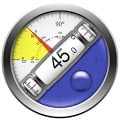# Clinometer + bubble level APK

This professional tool called Clinometer is the most precise (all you can get with some calculations) slope measurement tool for Android devices using all sides of the device plus the camera. It can be used for simple applications like aligning a frame as well as for more sophisticated fields of applications where an arbitrary slope needs to be measured exactly.
Common description of an inclinometer

A clinometer or inclinometer is an instrument for measuring angles of slope (or tilt), elevation or depression of an object with respect to gravity. It is also known as a tilt meter, tilt indicator, slope alert, slope gauge, gradient meter, gradiometer, level gauge, level meter, declinometer, and pitch & roll indicator. Clinometers measure both inclines (positive slopes, as seen by an observer looking upwards) and declines (negative slopes, as seen by an observer looking downward) using three different units of measure: degrees, percent, and topo (see Grade (slope). Astrolabes are inclinometers that were used for navigation and locating astronomical objects from ancient times to the Renaissance.
In aircraft, the "ball" in turn coordinators or turn and bank indicators is sometimes referred to as an inclinometer.

Drawing of an inclinometer, Museo Galileo, Florence.
Inclinometers include examples such as Well's in-clinometer, the essential parts of which are a flat side, or base, on which it stands, and a hollow disk just half filled with some heavy liquid. The glass face of the disk is surrounded by a graduated scale that marks the angle at which the surface of the liquid stands, with reference to the flat base. The zero line is parallel to the base, and when the liquid stands on that line, the flat side is horizontal; the 90 degree is perpendicular to the base, and when the liquid stands on that line, the flat side is perpendicular or plumb. Intervening angles are marked, and, with the aid of simple conversion tables, the instrument indicates the rate of fall per set distance of horizontal measurement, and set distance of the sloping line.
The Well's clinometer
The Abney level is a handheld surveying instrument developed in the 1870s that includes a sighting tube and inclinometer, arranged so that the surveyor may align the sighting tube (and its crosshair) with the reflection of the bubble in the spirit level of the inclinometer when the line of sight is at the angle set on the inclinometer.
One of the more famous inclinometer installations was on the panel of the Ryan NYP "The Spirit of St. Louis"—in 1927 Charles Lindbergh chose the lightweight Rieker Inc P-1057 Degree Inclinometer to give him climb and descent angle information.

Measuring slope with a clinometer
A forester using a clinometer makes use of basic trigonometry. First the observer measures a straight-line distance D from some observation point O to the object. Then, using the clinometer, the observer measures the angle a between O and the top of the object. Then the observer does the same for the angle b between O and the bottom of the object. Multiplying D by the tangent of a gives the height of the object above the observer, and by the tangent of b the depth of the object below the observer. Adding the two of course gives the total height (H) of the object.

The forester stands at a fixed distance from the base of the tree. The most common distances in the United States are 50 feet (15.24 m) and 66 feet (20.12 m). To obtain accurate readings it is best to use taped measured distance instead of paced distances. For the most accurate readings it is best to use a distance that is not less than the height of the tree being measured, that is, that the clinometer will measure an angle less than 45° (100%).

#### What’s New

✔ Fix inverted Camera with Nexus 5X and 6P

Name
Clinometer + bubble level
Package
com.plaincode.clinometer
Version
2.4
Size
15.22 MB
Installs